# WBJEE 2020 Physics Paper with Solutions

WBJEE 2020 Physics question paper and solutions are given on this page. BYJU’S provides step by step solutions that are prepared by experts. This has been done to address the need of students to find the right answer to each of the questions asked in the WBJEE 2020 Physics paper. Revising these solutions will help students to get an idea about the difficulty level and the question pattern of the exam. The PDF format of these solutions is also available for download at the bottom.

### WBJEE 2020 - Physics

Question 1: The bob of a swinging second's pendulum (one whose time period is 2s) has a small speed v0 at its lowest point. Its height from this lowest point, 2.25s after passing through it is

1. a. v02/2g
2. b. v02/g
3. c. v02/4g
4. d. gv02/4g

Solution:

Given: Time period of swinging seconds pendulum is 2s.

It means it completes its one revolution in 2s.

So the bob will reach its lowest point after 2s.

As it is travelling further for 1/4 sec. i.e. t = 2.25s

Hence, v = Aω cos ωt = v0 cos π/4

= v0/√2 { since Aω = v0 = vmax}

∵ displacement x = A sin ωt

Velocity, v = dx/dt = Aω cos ωt

From law of conservation of energy

½ mv02 = ½ m(v02/2)+mgh

½ v02 - ½ (v02/2) = gh

(1/2)v02(1-1/2) = gh

v02/4 = gh

⇒ h = v02/4

Question 2: A steel and brass wire, each of length 50 cm and cross-sectional area 0.005 cm2 hang from ceiling and are 15 cm apart. Lower ends of the wires are attached to a light horizontal bar. A suitable downward load is applied to the bar so that each of the wire extends in length by 0.1 cm. At what distance from the steel wire the load must be applied?

[Young's modulus of steel is 2×1012 dynes/cm2 and that of brass is 1×1012dynes/cm2]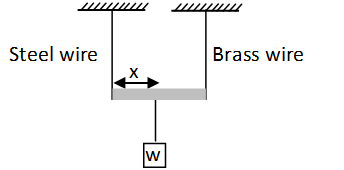1. a. 75 cm
2. b. 5 cm
3. c. 10 cm
4. d. 3 cm

Solution: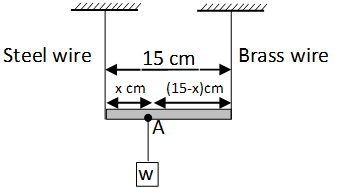Given:-

Length of steel and Brass wire each =50 cm.

Cross-sectional area = 0.005 cm2

Distance between the wires = 15 cm.

Extension in wire = 0.1 cm.

(Ys) Young's modulus of steel = 2×1012 dynes/cm2

(Yb) Young's modulus of brass = 1×1012 dynes/cm2

As we know that Young's modulus is given by

Y = stress/strain

Stress = force/area

Strain = change in length/original length

Y = [F/A]/[∆l/l]

⇒ F = YA∆l/l

∵ A, ∆l and l are same for both the wires.

Fs(x) = Fb(15-x)

Ys(x) = Yb(15-x)

Putting values of Ys and Yb

2×1012 (x) = (1×1012) (15-x)

2x = (15 – x)

3x = 15

x = 5cm.

Question 3: Which of the following diagrams correctly shows the relation between the terminal velocity VT of a spherical body falling in a liquid and viscosity v of the liquid?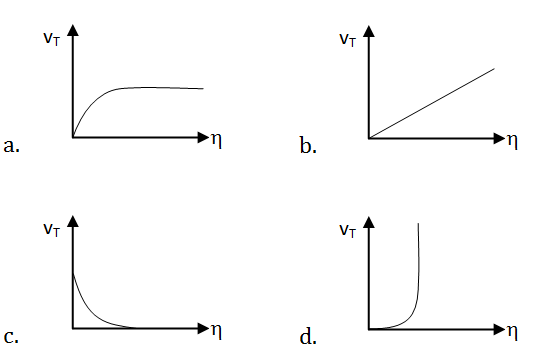Solution:

The force of viscosity acting on a smooth sphere in stream line motion can be expressed with Stock's formula -

F = 6 πηrv

Where F = force (N)

η = viscosity of fluid

r = radius of sphere (m)

v = relative velocity between fluid and sphere (m/s) or terminal velocity.

∴ v ∝ 1/η

Velocity decreases for each terminal velocity.

Question 4: An ideal gas undergoes the cyclic process abca as shown in the given P.V diagram. It rejects 50 J of the heat during ab and absorbs 80J of heat during ca. During bc, there is no transfer of heat and 40J of work is done by the gas. What should be the area of the closed curve abca?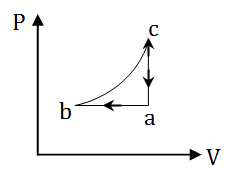1. a. 30 J
2. b. 40 J
3. c. 10 J
4. d. 90 J

Solution:

From P-V diagram (given)

a b = Isobaric process

c a = Isochoric process

It is a cyclic process and for cyclic process abca, the summation of heat will be equal to the summation of work.

Now ∆Q = Qab+Qbc+Qca

= -50+0+80

= 30J

{From Joule’s law, ∆W = ∆Q}

Question 5: A container AB in the shape of a rectangular parallelepiped of length 5m is divided internally by a movable partition P as shown in the figure. The left compartment is filled with a given mass of an ideal gas of molar mass 32 while the right compartment is filled with an equal mass of another ideal gas of molar mass 18 at the same temperature. What will be the distance of P from the left wall A when equilibrium is established?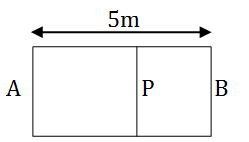1. a. 2.5 m
2. b. 1.8 m
3. c. 3.2 m
4. d. 2.1 m

Solution: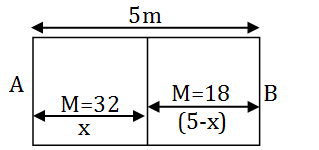For equilibrium pressure on both sides should be equal.

PV = nRT

Where P = Pressure {Since n = m/M }

n = no. of moles

V = volume

R = gas constant

T = Temperature

PV = (m/M)RT

P = mRT/MV

MV = Constant

At equilibrium, P1=P2 and T1=T2 (assuming wall to be conducting)

$$\frac{P_1V_1}{\mu_1}=\frac{P_1V_1}{\mu_2}=RT \(\frac{(5-x)32}{m}=\frac{18x}{m} x = 3.2 m Distance of the piston from A = 5-3.2 = 1.8 m Question 6: When 100 g of boiling water at 1000C is added into a calorimeter containing 300 g of cold water at 100C, temperature of the mixture becomes 200C. Then a metallic block of mass 1 kg at 100C is dipped into the mixture in the calorimeter. After reaching thermal equilibrium, the final temperature becomes 190C. What is the specific heat of the metal in C.G.S. units? 1. a. 0.01 2. b. 0.3 3. c. 0.09 4. d. 0.1 Solution: 1. Answer: (d) Initially By energy conservation, we can say that Heat loss by boiling water = heat gained by cold water Since Q = ms ∆ θ s = Specific heat capacity Q = heat ∆ θ = Temperature difference 100×1×80 = (300+W)1×10 Where W is the water equivalent of calorimeter ⇒ 8000 = 3000 + 10W ⇒ 5000 = 10W ⇒ W = 500 g When a block of mass 1 kg added at 100C then Total mass of water = 100 + 500 + 300 = 900 g 900×1×(20-19) = 1000×s ×(19-10) 9×1 = 10×s×9 1 = 10 s ⇒ s = 0.1 CGS unit Question 7: As shown in the figure, a point charge q1 = +1×10-6 C is placed at the origin in x-y plane and another point charge q2 = +3×10-6 is placed at the co-ordinate (10,0). In that case, which of the following graph(s) shown most correctly the electric field vector in Ex in x-direction?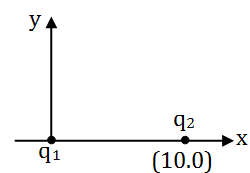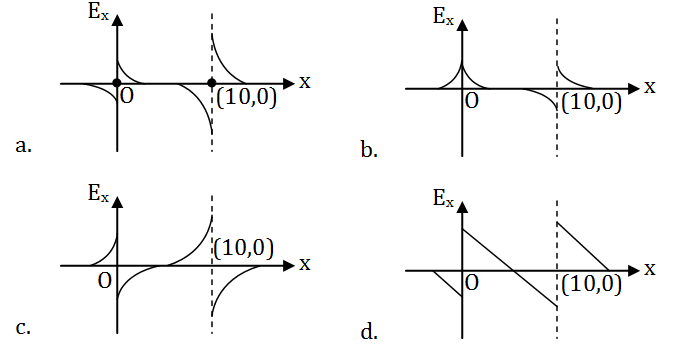Solution: 1. Answer: (a)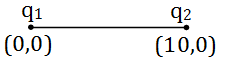q1 = 1×10-6 C q2 = 3×10-6 C Coulomb force between these charges will be F = kq1q2/r2 = 9x109x1 ×10-6 ×3 ×10-6/102 F = 27 ×10-5N Electric field, E = kq/r2 To the left of q1 resultant E1 + E2 will be in –ve x-direction To the right of q2 resultant E1 + E2 will be in +ve x-direction Also, this is a neutral point in between the charges as they are both positive charges.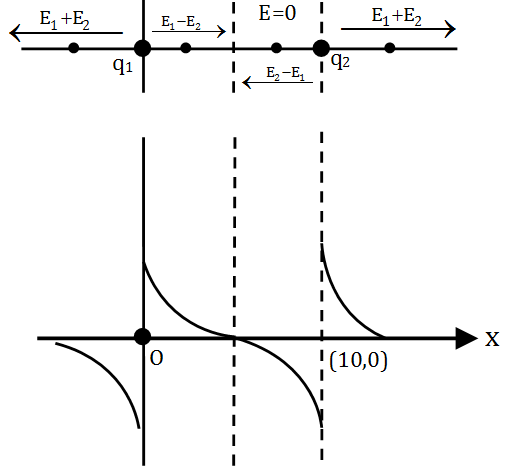Question 8: Four identical point masses, each of mass m and carrying charge +q are placed at the corners of a square of sides 'a' on a frictionless plane surface. If the particles are released simultaneously, the kinetic energy of the system when they are infinitely far apart is 1. a. (q2/4 π ε0a)(2√2+1) 2. b. (q2/4 π ε0a)(√2+2) 3. c. (q2/4 π ε0a)(√2+4) 4. d. (q2/4 π ε0a)(√2+1) Solution: 1. Answer: (c) From energy conservation (K.E)i+(P.E)i = (K.E)f +(P.E)f Since Electrostatic energy = kq2/r Here r = a Initial electrostatic energy = (1/4 π ε0) (q2/a)4+(q22)/ 4 π ε0a√2 = (q2/4 π ε0a)(4+√2) At infinity U = 0 (P.E. will be zero) ∆U = K.E. ⇒K.E. = (q2/4 π ε0a)(4+√2) It will be the kinetic energy of the system.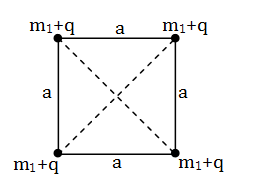Question 9: A very long charged solid cylinder of radius 'a' contains a uniform charge density ρ. The dielectric constant of the material of the cylinder is k. What will be the magnitude of the electric field at a radial distance 'x' (x < a) from the axis of the cylinder? 1. a. ρ(x/ε0) 2. b. ρ(x/2kε0) 3. c. ρ(x2/2aε0) 4. d. ρ(x/2k) Solution: 1. Answer: (b)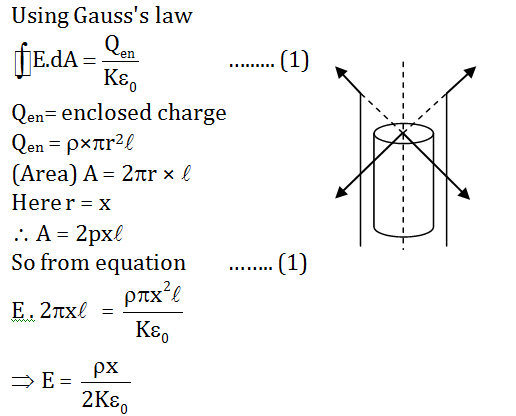Question 10: A galvanometer can be converted to a voltmeter of full-scale deflection V0 by connecting a series resistance R1 and can be converted to an ammeter of full - scale deflection I0 by connecting a shunt resistance R2. What is the current flowing through the galvanometer at its full-scale deflection? 1. a. V0-I0R2/(R1-R2) 2. b. V0+I0R2/(R1+R2) 3. c. V0-I0R1/(R1-R2) 4. d. V0+I0R1/(R1+R2) Solution: 1. Answer: (a) When galvanometer is used or converted as a voltmeter, resistance is used in series with it Ig(G+R1) = V0 G+R1 = V0/Ig V0 = Ig(G+R1) …(1) R1 = (V0/Ig)-G ….(A) When galvanometer is used or converted as an ammeter, the shunt is used in parallel with the galvanometer. Ig(G) = (I0-Ig)R2 R2 = IgG/(I0-Ig) …(2) G = R2(I0-Ig)/Ig …(3) From equation (1), (2) and (3) V = G Ig+R1Ig V = R2(I0-Ig)+R1Ig Ig = (V-I0R2 )/(R1-R2) Question 11: As shown in the figure a single conducting wire is bent to form a loop in the form of a circle of radius 'r' concentrically inside a square of side 'a', where a:r = 8 : π. A battery B drives a current through the wire. If the battery B and the gap G are of negligible sizes, determine the strength of the magnetic field at the common centre O.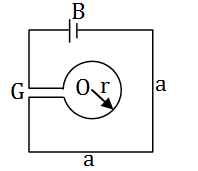1. a. (μ0I/2πa) √2(√2-1) 2. b. (μ0I/2πa)(√2+1) 3. c. (μ0I/πa)2√2(√2+1) 4. d. (μ0I/πa)2√2(√2-1) Solution: 1. Answer: (d) The magnetic field for square wire is given as; B = (μ0I/4πa)(sin α+sin β)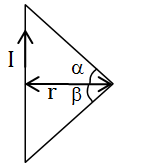r = a/2 B = (μ0I/4πa/2)(sin 450 +sin 450) For one-wire (out of the plane) B = (μ0I/4πa/2)(1/√2 +1/√2) B = (μ0I√2/2πa) For four wires, B1 = 4×(μ0I√2/2πa) B1 = (2√2 μ0I /πa) For circular loop, the magnetic field is given as B2 = (μ0I /2r) Given that a/r = 8/π ⇒ r = πa/8 B2 = (μ0I /2πa/8) = 4μ0I /πa (into the loop) (Into the plane) Net magnetic field B= B2-B1 B = (μ0I /πa)2√2(√2-1) Question 12: As shown in the figure, a wire is bent to a D-shaped closed-loop, carrying current I, where the curved part is a semi-circle of radius R. The loop is placed in a uniform magnetic field B, which is directed into the plane of the paper. The magnetic force felt by the closed-loop is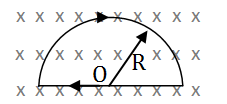1. a. 0 2. b. IRB 3. c. 2IRB 4. d. (1/2)IRB Solution: 1. Answer: (a) The figure forms a closed loop and the current completes the loop. Therefore, the net force on the loop in the uniform field should be zero. Question 13: What will be the equivalent resistance between the terminals A and B of the infinite resistive network shown in the figure?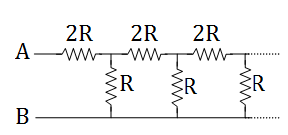1. a. (√3+1)/2 2. b. (√3-R)/2 3. c. 3R/2 4. d. (√3+1)R Solution: 1. Answer: (d) Equivalent circuit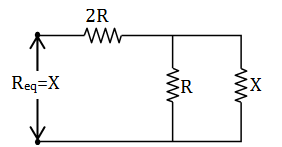From the figure, we can see that resistance x and R in parallel and then connected with R in series. So X = [RX/(R+X)]+2R X = (RX+2R(R+X)/(R+X)) X(R+X) = RX+2R2+2RX XR+X2 = RX+2R2+2RX X2-2RX-2R2 = 0 ⇒ X = (1+√3)R Question 14: When a DC voltage is applied at the two ends of a circuit kept in a closed box, it is observed that the current gradually increases from zero to a certain value and then remains constant. What do you think that the circuit contains?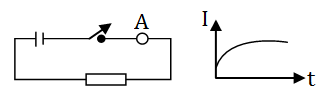1. a. A resistor alone 2. b. A capacitor alone 3. c. A resistor and an inductor in series 4. d. A resistor and a capacitor in series Solution: 1. Answer: (c) According to the graph, it is the condition of steady-state. In this case, the current gradually increases from zero to a certain value and then remains constant. It will occur in LR circuit transient. In steady-state, a capacitor behaves like an infinite resistance while an inductor will behave like a wire. Hence, the current is constant with the inductor and zero with the capacitor. Since DC current is given, that's why resistance in series is also necessary. Question 15: Consider the circuit shown. If all the cells have negligible internal resistance, what will be the current through the 2 ohm resistor when steady state is reached?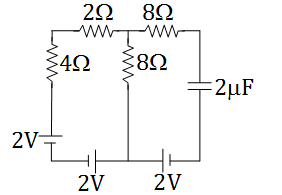1. a. 0.66 A 2. b. 0.29 A 3. c. 0 A 4. d. 0.14 A Solution: 1. Answer: (c) The equivalent emf of loop = 0 The capacitor is in steady-state and in this state the capacitor draws no current from the cell. So the circuit is open. Hence no current passes through it.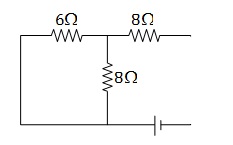Question 16: Consider a conducting wire of length L bent in the form of a circle of radius R and another conductor of length 'a' (a<<R) bent in the form of a square. The two loops are then placed in the same plane such that the square loop is exactly at the centre of the circular loop. What will be the mutual inductance between the two loops? 1. a. μ0 πa2/L 2. b. μ0 πa2/16 L 3. c. μ0 πa2/4L 4. d. μ0a2/4πL Solution: 1. Answer: (b) Let i be the current flowing through the circular loop. Magnetic field due to a circular loop at the centre.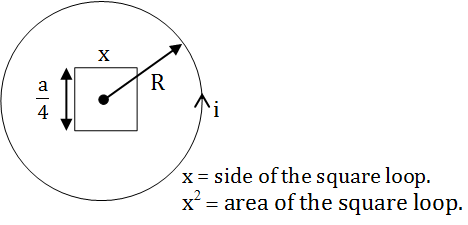B = μ0i/2R …..(1) Circumference of the loop L = 2πR R = L/2π ….(2) Now flux through the square loop φ = BA A = area of loop = x2 φ = B(x2) …(3) Given x = a/4 …(4) We know that, φ = Mi, where φ = flux, M = Mutual inductance, i = current Mi = Bx2 ………… (5) Putting the values from equation (1), (2), (4) in equation (5)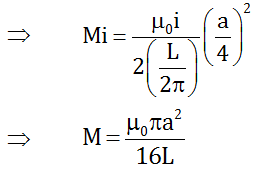Question 17: An object is placed 60 cm in front of a convex mirror of focal length 30 cm. A plane mirror is now placed facing the object in between the object and the convex mirror such that it covers the lower half of the convex mirror. What should be the distance of the plane mirror from the object so that there will be no parallax between the images formed by the two mirrors? 1. a. 40 cm. 2. b. 30 cm. 3. c. 20 cm. 4. d. 15 cm. Solution: 1. Answer: (a)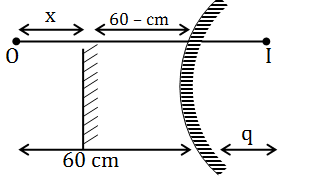Let light is incident from left to right Given focal length of convex mirror (f) = 30 cm. Object distance u = 60 cm Image distance = (v) = q From mirror formula 1/f = (1/v)+(1/u) …(1) f = 30 cm. (+ve) u = -60 (taking direction is opposite to the incident light) v = q (+ve) (distance of the image, as we know convex mirror always forms virtual erect and diminished image) Substituting the values in equation (1) 1/30 = (1/q)-(1/60) 1/q = (1/30)+(1/60) = (2+1)/60 q = 60/3 = 20 cm Plane mirror forms an image at the same distance as the object is placed. Hence, for the condition of no parallax. x = (60-x) + q x = 60-x + 20 x = 40 cm Question 18: A thin convex lens is placed just above an empty vessel of depth 80 cm. The image of a coin kept at the bottom of the vessel is thus formed 20 cm above the lens. If now, water is poured in the vessel up to a height of 64 cm, what will be the approximate new position of the image? Assume that the refractive index of water is 4/3. 1. a. 21.33 cm above the lens 2. b. 6.67 cm below the lens 3. c. 33.67 cm above the lens 4. d. 24 cm above the lens Solution: 1. Answer: (a) Using the lens formula 1/f = (1/v)-(1/u) For convex lens Focal length f = +ve Object distance u = –ve (∵ +ve and –ve sign considered according to the direction of incident ray) So, (1/v)-(1/u) = 1/f (1/20)+(1/80) = 1/f 1/f = (4+1)/80 f = 16 cm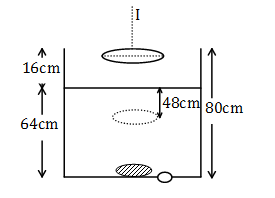Now when water is poured, the image will shift. Its distance from the surface of water h' = h/μ = [64 * 3] / 4 = 48cm Hence its distance from lens = 48+16 = 64 cm This will be the new object distance. Now we have to calculate the value of v, again using lens formula (1/v)-(1/-64) = 1/16 1/v = (1/16)-(1/64) 1/v = (4-1)/64 v = 64/3 = 21.33 cm above the lens Question 19: The intensity of light emerging from one of the slits in Young's double-slit experiment is found to be 1.5 times the intensity of light emerging from the other slit. What will be the approximate ratio of the intensity of an interference maximum to that of an interference minimum? 1. a. 2.25 2. b. 98 3. c. 5 4. d. 9.9 Solution: 1. Answer: (b) I1 = 1.5I2 I1/I2 = 1.5 I1/I2 = 3/2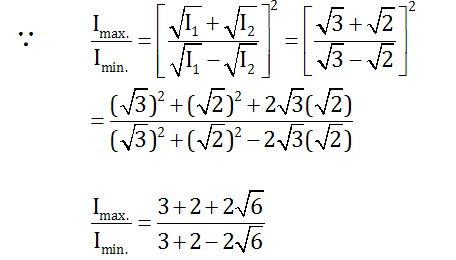= (5+2√6)/(5-2√6) = (5+2√6)2/(52 -(2√6)2) = (25+24+20√6)/(25-24) = 97.8 ≈ 98 Question 20: In a Fraunhofer diffraction experiment, a single slit of width 0.5 mm is illuminated by a monochromatic light of wavelength 600 nm. The diffraction pattern is observed on a screen at a distance of 50 cm from the slit. What will be the linear separation of the first order minima? 1. a. 1.0 mm 2. b. 1.1 mm 3. c. 0.6 mm 4. d. 1.2 mm Solution: 1. Answer: (d) Given d = 0.5 mm, D = 50 cm, λ = 600 nm Let x be the distance between the first order minima's x = 2 λD/d Substituting the values x = 2x600x10-9x50x10-2/(0.5x10-3) = 12x10-4 x = 1.2 mm Question 21: If R is the Rydberg constant in cm-1, then hydrogen atom does not emit any radiation wavelength in the range of 1. a. 1/R to 4/3R cm 2. b. 7/5R to 19/5R cm 3. c. 4/R to 36/5R cm 4. d. 9/R to 144/7R cm Solution: 1. Answer: (b) As we know that the wave-number is given by 1/ λ = R[1/nf2 - 1/ni2] For the range of wavelength:- ni = 1,2,3 ….for Lyman, Balmer, Paschen nf = ni +1 and nf = ∞ for upper and lower range Thus, Lyman: [1/R to 4/3R cm] Balmer: [4/R to 36/5R cm] Paschen: [9/R to 144/36R cm] Bracket: [ 16/R to 400/9R cm] Pfund: [25/R to 900/11R cm] So Option A belongs to Lyman series Option C belongs to Balmer series Option D belongs to Paschen series Option B does not belong to any transition series. Therefore hydrogen atom does not emit any radiation of wavelength in this range. Question 22: A nucleus X emits a beta particle to produce a nucleus Y. If their atomic masses are Mx and My respectively. The maximum energy of the beta particle emitted is (where me is the mass of an electron and c is the velocity of light) 1. a. (Mx-My-me)c2 2. b. (Mx-My+me)c2 3. c. (Mx-My)c2 4. d. (Mx-My+2me)c2 Solution: 1. Answer: (c)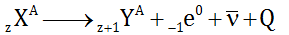Let Mx be the mass of the nucleus X and My be the mass of the nucleus Y. Since mass change= Mx-My This will be the mass reduced to β-particle. Hence, energy E = ∆mc2 E = (Mx-My)c2 Question 23: For nuclei with a mass number close to 119 and 238, the binding energies per nucleon are approximately 7.6 MeV and 8.6 respectively. If a nucleus of mass number 238 breaks into two nuclei of nearly equal masses, what will be the approximate amount of energy released in the process of fission? 1. a. 214 MeV 2. b. 119 MeV 3. c. 2047 MeV 4. d. 1142 MeV Solution: 1. Answer: (a) Energy released = Total binding energy of reactant – Total binding energy of products. E = 238 × 8.6 – 119×2×7.6 E = 238 ×(1) (MeV) 119 -> 7.6 MeV 238 -> 119+119 Since the energy released will also be transferred as kinetic energy of the daughter nuclei. Therefore the answer closest to 238 MeV from option should be chosen. Question 24: A common emitter transistor amplifier is connected with a load resistance of 6-kilo ohm. When a small a.c. signal of 15 mV is added to the base-emitter voltage, the alternating base current is 20μA and the alternating collector current is 1.8 mA. What is the voltage gain of the amplifier? 1. a. 90 2. b. 640 3. c. 900 4. d. 720 Solution: 1. Answer: (d) Load resistance RL = 6 kilo ohm = 6000 ohm Base emitter voltage VBE = 15 mV = 15×10-3V Base current Ib = 20μA = 20×10-6A Collector current Ic= 1.8 mA = 1.8×10-3 A Input resistance R = VBE/IB = 15x10-3/20x10-6 = (3/4)1000 Current gain , β = ∆Ic/∆Ib = 1.8x10-3/20x10-6 = 90 ∵Voltage gain (Av) = βRL/R1 = 90x6000x4/3x1000 = 720 Question 25: In the circuit shown, the value of β of the transistor is 48. If the base current supplied 200 μA, what is the voltage at the terminal Y?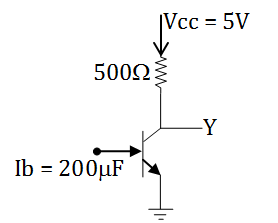1. a. 0.2 V 2. b. 0.5 V 3. c. 4 V 4. d. 4.8 V Solution: 1. Answer: (a) β = 48 (given)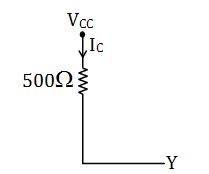∵ IB = 200μA = 200x10-6A β = Ic/Ib βIB = 48x200x10-6 = 96x10-4A Now VCC = ICIR +VCE ∴ VCE = 5 – (96×10-4)×500 (Voltage at terminal Y) Vy = VCE = 5-4.8 = 0.2 volt Question 26: The frequency v of the radiation emitted by an atom when an electron jumps from one orbit to another is given by v = kδE, where k is a constant and δE is the change in energy level due to the transition. Then dimension of k is 1. a. ML2T-2 2. b. The same dimension of angular momentum. 3. c. ML2T-1 4. d. M-1L-2T Solution: 1. Answer: (d) v = kδE k = v/δE = [T-1 ]/[M1L2T-2] = [M-1L-2T1] Dimension of frequency = 1/sec = [T-1] Dimension of energy = [M1L2T-2] Question 27: Consider the vectors \(\vec{A}= \hat{i}+\hat{j}-\hat{k}$$, $$\vec{B}= \hat{2i}-\hat{j}+\hat{k}$$, $$\vec{C}=\frac{1}{\sqrt{5}}(\hat{i}-2\hat{j}+2\hat{k})$$. What is the value of $$\vec{C}.(\vec{A}\times \vec{B})$$?

1. a. 1
2. b. 0
3. c. 3√2
4. d. 18√5

Solution: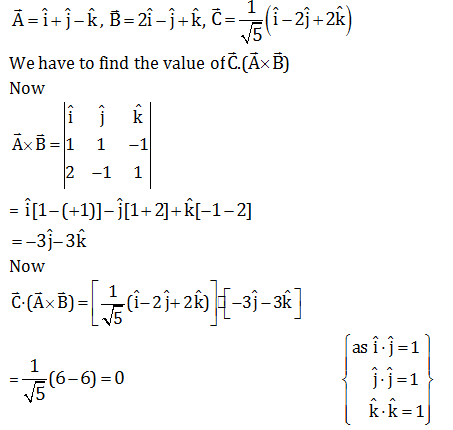Question 28: A fighter plane, flying horizontally with a speed of 360 km/h at an altitude of 500 m drops a bomb for a target straight ahead of it on the ground. At what approximate distance, the bomb should be dropped ahead of the target, so that it hits the target? Assume that acceleration due to gravity g is 10 ms-2. Also, neglect air drag.

1. a. 1000 m
2. b. 50√5 m
3. c. 500√5 m
4. d. 866 m

Solution:

u = 360 km/hr

= 360 ×5/18 = 100 m/s

h = 500 m

Since R = u√(2h/g)

R = 100√(2×500/10)

R = 100 ×10

R = 1000 m

Question 29: A block of mass m rests on a horizontal table with a coefficient of static friction μ. What minimum force must be applied on the block to drag it on the table?

1. a. μ/√(1+μ2) mg
2. b. (μ-1)/(μ +1) mg
3. c. μ/√(1-μ2) mg
4. d. μmg

Solution: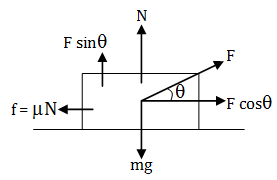f = friction force

F cos θ = horizontal component of applied force

F sin θ = vertical component of applied force.

Now applying equilibrium in vertical direction

F sin θ+N = mg …… (1)

N = mg - F sin θ…… (2)

Applying equilibrium in horizontal direction

F cos θ = μN

F cos θ = μ(mg-F sin θ) {from equation (2)}

F cos θ+ μ F sin θ = μ mg

F (cos θ + μ sin θ ) = μ mg

F = μ mg/(cos θ + μ sin θ )…… (3)

For F minimum, (cos θ + μ sin θ) should be maximum

∴ (d/dθ) (cos θ + μ sin θ) = 0

-sin θ+ μ cos θ = 0

μ cos θ = sin θ

μ = tan θ …… (4)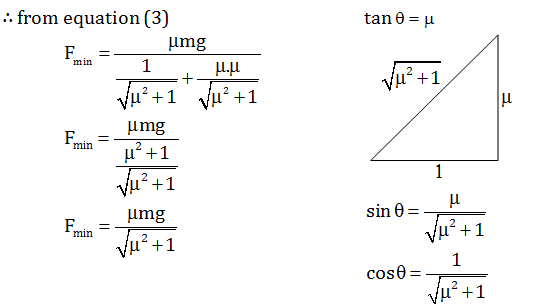Question 30: A tennis ball hits the floor with a speed v at an angle θ with the normal to the floor. If the collision is inelastic and the coefficient of restitution is ε, what will be the angle of reflection?

1. a. tan-1 [(tan θ)/ ε]
2. b. sin-1 [(sin θ)/ ε]
3. c. θε
4. d. θ(2ε)/ε+1

Solution:

The velocity of approach in the given case is the normal component of velocity.

Hence vn = v cos θ

By definition of coefficient of restitution, velocity of separation will be,

v'n = ∈vn = ∈v cos θ

Tangential component of velocity will not change

v't = vt = v sin θ

Speed of reflected ball is

v' = √(vn2+vt2)

= √[(∈ v cos θ)2+(v sin θ)2]

= v√( ∈2cos2 θ + sin2 θ)

Angle with normal is given by

θ' = tan-1 (v't / v'n)

= tan-1 (v sin θ/ ∈v cos θ)

= tan-1 [(tan θ)/ ∈]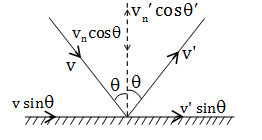Question 31: A metallic block of mass 20 kg is dragged with a uniform velocity of 0.5 ms-1 on a horizontal table for 2.1 s. The coefficient of static friction between the block and the table is 0.10. When will be the maximum possible rise in temperature of the metal block, if the specific heat of the block is 0.1 C.G.S.unit? Assume g = 10 ms-1and uniform rise in temperature throughout the whole block. [Ignore absorption of heat by the table]

1. a. 0.00250C
2. b. 0.00350C
3. c. 0.0010C
4. d. 0.050C

Solution:

Mass , m = 20 kg

Velocity, v = 0.5 ms-1

Time = 2.1s

μ = 0.10

Distance or displacement

s = v × t = 0.5×2.1

s = 1.05 m.

Friction force

F = μmg

F = 0.1×20×10 = 20

∴Work done= F.s

W = 20 × 1.05 = 21 J

This work done by friction is converted into heat energy.

W = Q

W = ms∆T

∴Q = ms∆T

21 = 20×0.1×4.2×103×∆T

∆T = 21/(20x0.1x4.2x103)

∆T = 0.00250C

Question 32: Consider an engine that absorbs 130 cal of heat from a hot reservoir and delivers 30 cal heat to a cold reservoir in each cycle. The engine also consumes 2J energy in each cycle to overcome friction. If the engine works at 90 cycles per minute, what will be the maximum power delivered to the load?

1. a. 816 W
2. b. 819 W
3. c. 627 W
4. d. 630 W

Solution:

Absorbed heat = 130 Calorie from hot reservoir

Delivered heat = 30 Calorie to cold reservoir

Consume heat = 2J energy in each cycle

The engine works at 90 cycles per minute.

Work done per cycle = (130-30)4.2-2 {1 Calorie = 4.2 Joule}

= 100×4.2-2

= 420-2 = 418 J

Total work done = 418×90

{∵ In each cycle work done = 418 J}

Power = work done/time

P = W/t = 418x90/60

= 418x3/2

= 627 W

Question 33: Two pith balls, each carrying charge +q are hung from a hook by two strings. It is found that when each charge is tripled, angle between the strings double. What was the initial angle between the strings?

1. a. 300
2. b. 600
3. c. 450
4. d. 900

Solution:

In first case

Applying equilibrium in horizontal direction

T sin θ = F… (1)

Applying equilibrium in vertical direction

T cos θ = mg ……. (2)

Divide (1) by (2)

tan θ = F/mg …… (3)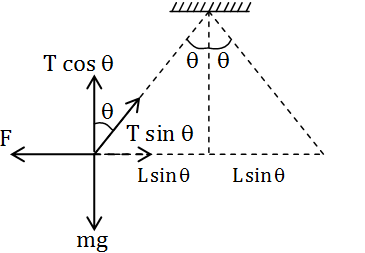tan θ = (kq2/2L sin θ)mg …… (4) { Since F = kq1q2/r2}

In second case, when each charge is tripled then q becomes 3q

tan 2θ = (kq q2/(2L sin θ)2 mg) …… (5)

Equation (5) divided by equation (4)

tan 2θ/tan θ = 9 sin2 θ/sin2

2/1-tan2 θ = 9/4 cos2 θ

= 9/4 sec2 θ

2/1-tan2 θ = (9/4)(1-tan2 θ)

Put tan2 θ = x

So 2/1-x = (9/4)(1+x)

8 = 9-9x2

⇒ x = 1/3

⇒ tan2 θ = 1/3

⇒ θ = 300

Initial angle between the strings = 300

If it would have been asked final answer then angle would have been = 600

Question 34: A conducting circular loop of resistance 20 ohm and cross sectional area 20×10-2 m2 is placed perpendicular to a spatially uniform magnetic field B, which varies with time t as B = 2 sin(50 πt) T. Find the net charge flowing through the loop in 20 ms starting from t = 0.

1. a. 0.5 C
2. b. 0.2 C
3. c. 0 C
4. d. 0.14 C

Solution:

Given

R = 20 ohm

A(area) = 20×10-2 m2,

Magnetic field = B varies with time as B = 2 sin (50πt)T

q = ?

time (t) = 20ms = 20×10-3s.

Initial time t = 0

∵ Magnetic flux, φ = B.A

emf , ε = dφ/dt

= A(dB/dt)

= A(d/dt)(2 sin (50πt)T

ε = A × 100π cos (50 π t)…… (1)

since current I = dq/dt = ε/R

dq = (ε/R)dt …..(2)

From equation (1) and (2)

dq = (A/R)[100π cos (50 π t)]dt……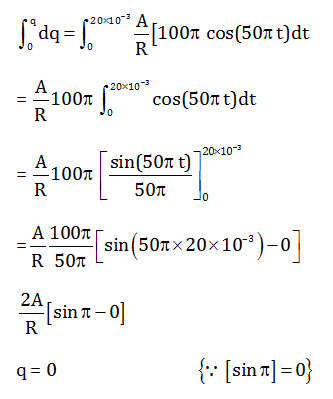Question 35: A pair of parallel metal plates is kept with a separation, d. One plate is at a potential +V and the other is at ground potential. A narrow beam of electrons enters the space between the plates with a velocity v0 and in a direction parallel to the plates. What will be the angle of the beam with the plates after it travels an axial distance L?

1. a. tan-1[eVL/mdv0]
2. b. tan-1[eVL/mdv02]
3. c. sin-1 [eVL/mdv0]
4. d. sin-1[eVL/mdv02]

Solution:

Since time = distance /speed

t = L/v0 …(1)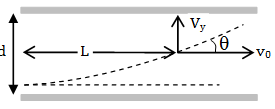v0 = velocity component along x-axis

vy = velocity component along y-axis

∵F = ma

F = qE = ma {since F = qE}

Here q = e

⇒ a = eE/m

Since a = v/t

v = at

so v = eEt/m

Substituting the value of t from equation (1)

vy = eVL/mdv0 [ since E = V/d]

tan θ = velocity component of y/ velocity component of x

= vy/vx = (e/m)(V/d)(L/v0)(1/v0)

tan θ = eVL/mdv02

θ = tan-1 (eVL/mdv02)

Question 36: A simple pendulum of length l is displaced so that its taut string is horizontal and then released. A uniform bar pivoted at one end is simultaneously released from its horizontal position. If their motions are synchronous, what is the length of the bar?

1. a. 3l/2
2. b. l
3. c. 2l
4. d. 2l/3

Solution:

The time period of a simple pendulum;

T = 2 π √(l/g)

l = length of the string

g = acceleration due to gravity

For a compound pendulum, let L' be the length, I be the moment of inertia about the pivot.

So time period, T’ = 2π√(I/Mgd)

$$=2\pi \sqrt{\frac{ML^{'2}}{3Mg\frac{L'}{2}}}$$

where d = 3L’/2

T’ = 2π√(2L’/3g)

Since motion is synchronous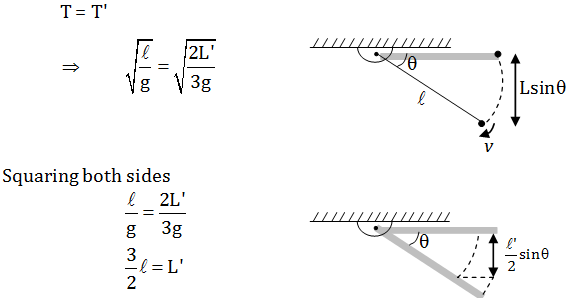Question 37: A charged particle moves with constant velocity in a region where no effect of gravity is felt but an electrostatic field $$\vec{E}$$ together with a magnetic field $$\vec{B}$$ may be present. Then which of the following cases are possible?

1. a. $$\vec{E}$$ ≠ 0, $$\vec{B}$$ ≠ 0
2. b. $$\vec{E}$$ ≠ 0, $$\vec{B}$$ = 0
3. c. $$\vec{E}$$ = 0, $$\vec{B}$$ = 0
4. d. $$\vec{E}$$ = 0, $$\vec{B}$$ ≠ 0

Solution:

If a charged particle moves in a gravity free space without change in velocity then,

(1) Particle can move with constant velocity in any direction, so B = 0, E = 0

(2) Particle can move in a circle with constant speed. The magnetic force will provide the centripetal force that causes the particle to move in a circle.

(3) If qE = qvB and magnetic and electric force in opposite direction, in this case also particle move with uniform speed.

Question 38: A 400 ohm resistor, a 250mH inductor and a 2.5 μF capacitor are connected in series with AC source of peak voltage 5V and angular frequency 2kHz. What is the peak value of the electrostatic energy of the capacitor?

1. a. 2 μJ
2. b. 2.5J μ
3. c. 3.3 μJ
4. d. 5 μJ

Solution: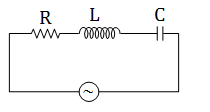Given values

R = 40 ohm

L = 250 mH

C = 2.5 μF

VP = 5V

ω = 2KHz

Inductive reactance, XL = ωL

= 2×103×250×10-3 ohm

XL = 500 ohm

Capacitive reactance, Xc = 1/ωC

= 1/ 2x103x2.5x10-6

= 200 ohm

Impedance, Z = √(R2+(XL-XC)2)

= √(400)2+(500-200)2

= 500 ohm

Peak current, IP = VP/Z = 5/500

= 1/100 A

∴Voltage (Peak) drop in C

VC = IPXC

= (1/100)200

= 2V

∴ Peak electrostatic energy of the capacitor

EC = (½)CV2

= (1/2)2.5x10-6x22

= 5μJ

Question 39: A point source of light is used in an experiment of photoelectric effects. If the distance between the source and the photo-electric surface is doubled, which of the following may result?

1. a. Stopping potential will be halved
2. b. Photoelectric current will decrease
3. c. Maximum kinetic energy of photo-electron will decrease
4. d. Stopping potential will increase slightly

Solution:

∵ Intensity, I ∝ 1/d2

Where d = distance between the source and surface.

So if the distance between the source and surface is doubled i.e. d' = 2d, then the intensity of light falling on the surface becomes one fourth

i.e., I’ = 1/(2d)2 = (¼)I

and photoelectric current ∝ intensity

∴ current will decrease

The stopping potential (VS) in the photoelectric effect is related to the incident light frequency (v) by the following equation.

eVs = hv- φ, where φ is the work function of the material. It does not depend on the distance of the light source from the emitting metal. So, the stopping potential will remain constant in this case.

Question 40: Two metallic spheres of equal outer radii are found to have same moment of inertia about their respective diameters. Then which of the following statement(s) is/are true?

1. a. Two spheres have equal mass
2. b. The ratio of masses is nearly 1.67 : 1
3. c. The spheres are made of different materials
4. d. Their rotational kinetic energies will be equal when rotated with equal uniform angular speed about their respective diameters

Solution:

To calculate kinetic energy when both the spheres are rotated with equal uniform angular speed about their diameters it will be given by

K.E = (½)I ω2

∵ according to the question in option (d), ω is given the same for both the spheres. In question, it has already been mentioned that moment of inertia about their diameters is same. So we can say that their rotational kinetic energy will be constant.

As only the outer radius is given same, so masses need not be equal as the change in inner radius can cancel out the effect of change in mass to maintain the same moment of inertia of both spheres.

As we already mentioned that in question only outer radius is given so to determine the ratio of mass, the inner radius is also required.

We can't comment on the density of the sphere because information about inner radius not given.

### WBJEE 2020 Physics Question Paper with Solutions